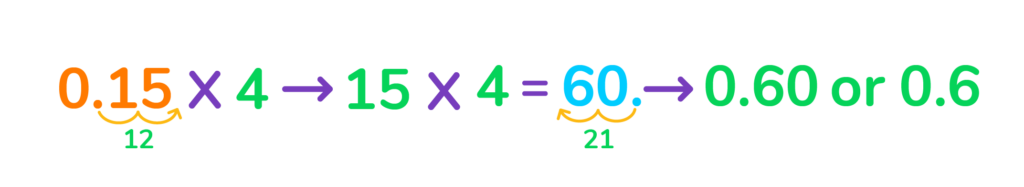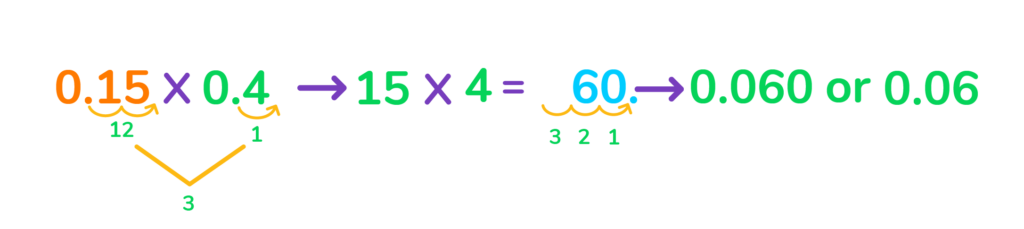# How to Multiply Decimals

All you need to know about multiplying decimals by each other, whole numbers, and exponents. Boost your skills and confidence with this step-by-step guide.Author
Tess LouckaPublished
November 7, 2023# How to Multiply Decimals

All you need to know about multiplying decimals by each other, whole numbers, and exponents. Boost your skills and confidence with this step-by-step guide.Author
Tess LouckaPublished
Nov 1, 2023# How to Multiply Decimals

All you need to know about multiplying decimals by each other, whole numbers, and exponents. Boost your skills and confidence with this step-by-step guide. them to real problemsAuthor
Tess LouckaPublished
Nov 7, 2023Key takeaways

• Knowing how to multiply decimals is a crucial skill that you’ll use throughout your daily life.
• To multiply decimals, convert them into whole numbers first. Multiply the whole numbers together, then place the decimal points back so the final answer has the same number of decimal spaces as the two factors.
• When multiplying decimals by 10, 100, 1,000, or other exponents, move the decimal point to the right the same number of spaces as there are zeros.

Multiplying decimals is a simple math calculation that you’ll be using for the rest of your life. Calculating money, time, weight, length, and distance are all likely to involve decimals, and knowing how to multiply decimals confidently will save you plenty of trouble and time in the future.

For example, you multiply decimals when calculating how much gas you can afford at a rate of \$3.67 per gallon, or how far you can walk at a rate of 2.5 miles per hour.

Decimal multiplication is easy once you know the trick!

## How to Multiply Decimals Step by StepThe easiest way to multiply decimals is to convert them to whole numbers first, multiply them, then convert them back to decimals.

To explain how to multiply with decimals using this technique, let’s look at a few methods with examples.

### Multiplying decimals by whole numbersTo start, let’s learn how to multiply decimals by whole numbers.

Take 0.15 x 4 as an example.

Step 1: To solve this problem, let’s turn the decimal number into a whole number by moving the decimal point to the right two places so that it falls after the digits.In this example, 0.15 becomes 15 when we move the decimal point over 2 spaces.

Step 2: Now, multiply 15 x 4 to get 60.

Step 3: Next, convert this number back into a decimal number. Since we moved the decimal point over two spaces to the right in Step 1, we now have to move the decimal point over two spaces to the left.

The final answer is 0.15 x 4 = 0.60 or 0.6

### Multiplying two decimalsNow let’s take a look at how we can multiply two decimal numbers together.

We will use 0.15 x 0.4 as our example.

Step 1: First, turn both numbers into whole numbers by moving their decimal points to the right so they fall after the digits.Step 2: Next, we multiply our new factors, 15 and 4, to get 60.

Step 3: Lastly, convert the whole numbers back into decimals. We moved the decimal point over two spaces to the right for our first number, 0.15, and one space to the right for our second number, 0.4. That’s three spaces in total.

So, we have to move the decimal space back to the left by three spaces to get our final answer.

0.15 x 0.4 = 0.060 or 0.06

### Multiplying decimals by 10, 100, and 1,000To multiply a decimal by 10, 100, or 1,000, all we have to do is move the decimal point over to the right the same number of spaces as there are zeros.

Let’s look at an example:

11.7 x 10 = 117

There is only one zero in 10, so we move the decimal point over to the right by one to get our answer.

But how would we multiply this number by 100?

11.7 x 100 = 1,170

In this case, we have to move the decimal point over to the right by two spaces because there are two zeros in 100. That means we’ll have to move the decimal point a space past the 7. We can fill in that space with a 0.

11.7 x 1,000 is 11,700

Move the decimal point to the right by three spaces since there are three zeros in 1,000. The empty spaces can be replaced with zeros.

For more practice multiplying with decimals, download a math practice app or use a math website that offers practice problems, answers, and explanations to all your questions. The more often you practice multiplying decimals, the more confident you’ll be.

## Practice problems

Click on the boxes below to see the answers!

Turn both numbers into whole numbers. Solve for 145 x 13 to get 1,885. Next, add the decimal points back in. You moved the decimal point to the right two spaces for 1.45 and two spaces for 0.13 to turn them into whole numbers, so move the decimal point to the left by 4 spaces for the final answer. That means 1,885 turns into 0.1885.

Write out your equation for the problem, 1.25 x 12. Turn 1.25 into a whole number. Solve for 125 x 12 to get 1,500. Then, add the decimal points back in by counting two spaces to the left. So, 1,500 becomes 15.00 or 15. Use a dollar sign since the problem refers to money.

When multiplying by 10, 100, or 1,000, move the decimal point to the right the same number of spaces as there are zeros. Since 100 has two zeros, move the decimal point to the right by two. So, 1.4 becomes 140.Turn the decimal number into a whole number. Multiply the whole numbers together, then add the decimal point back in to get your final answer.

Turn both numbers into whole numbers and multiply. Put the decimal points back in by counting the decimal spaces you got rid of when converting them into whole numbers. There should be the same number of decimal spaces in the final answer.

Turn them into whole numbers, multiply them, and convert them back to decimals.

Three steps. Step 1: Make the decimal numbers whole numbers. Step 2: Multiply the whole numbers. Step 3: Add the decimal points back in.Lesson credits

Tess Loucka

Tess Loucka discovered her passion for writing in high school and has not stopped writing since. Combined with her love of numbers, she became a math and English tutor, focusing on middle- and high-school-level topics. Since graduating from Hunter College, her goal has been to use her writing to spread knowledge and the joy of learning to readers of all ages.

Tess Loucka

Tess Loucka discovered her passion for writing in high school and has not stopped writing since. Combined with her love of numbers, she became a math and English tutor, focusing on middle- and high-school-level topics. Since graduating from Hunter College, her goal has been to use her writing to spread knowledge and the joy of learning to readers of all ages.# Are you a parent, teacher or student?

Are you a parent or teacher?

## Hi there!

Book a chat with our team